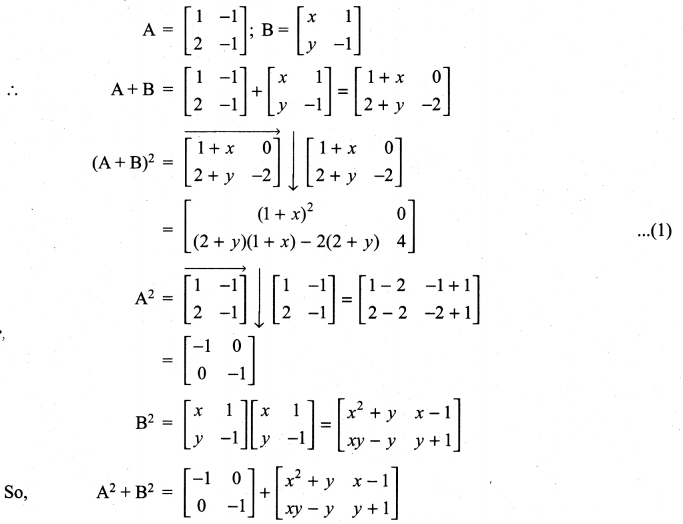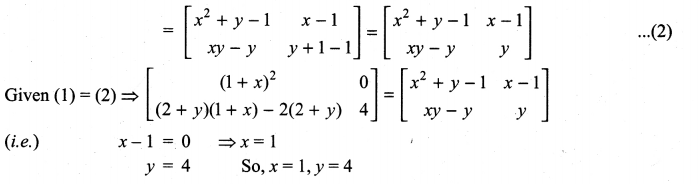## Tamilnadu Samacheer Kalvi 11th Maths Solutions Chapter 7 Matrices and Determinants Ex 7.1

11th Maths Exercise 7.1 Answers Question 1.
Construct an m × n matrix A = [aij], where aij is given by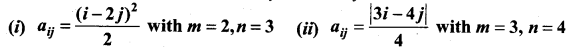Solution:
(i) aij = $$\frac{(i-2 j)^{2}}{2}$$
Here m = 2, n = 3
So we have to construct a matrix of order 2 × 3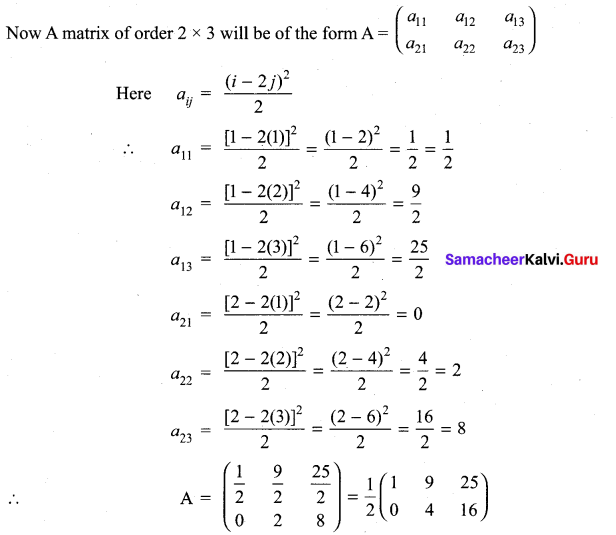(ii) Here m = 3 and n = 4
So we have to construct a matrix order 3 × 4
The general form of a matrix of order 3 × 4 will be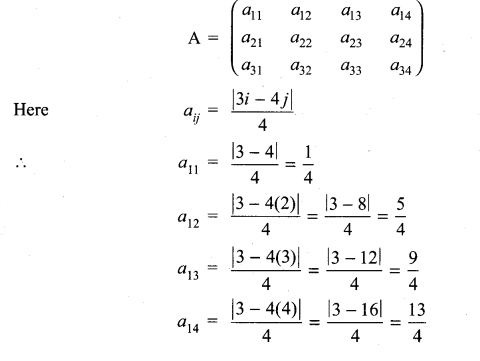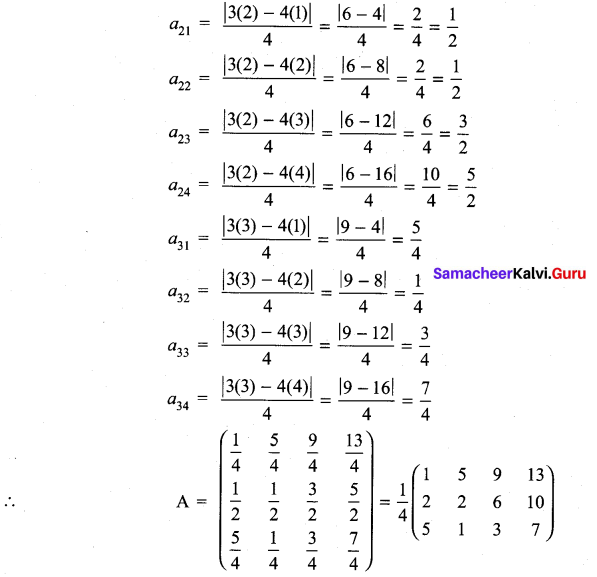11th Maths Exercise 7.1 Question 2.
Find the values of p, q, r and s if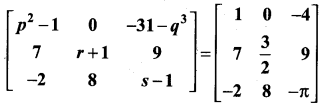Solution:
When two matrices (of same order) are equal then their corresponding entries are equal.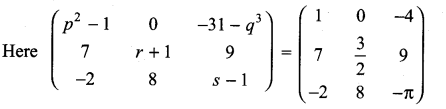⇒ p2 – 1 = 1
⇒ p2 = 1 + 1 = 2
p = ± $$\sqrt{2}$$
-31 – q3 = -4
-q3 = -4 + 31 = 27
q3 = -27 = (-3)3
⇒ q = -3
r + 1 = $$\frac{3}{2}$$
⇒ r = $$\frac{3}{2}$$ – 1 = $$\frac{3-2}{2}$$ = $$\frac{1}{2}$$
s – 1 = π
⇒ s = – π + 1 (i.e.,) s = 1 – π
So, p = ± $$\sqrt{2}$$, q = -3, r = 1/2 and s = 1 – π

11th Maths Matrices And Determinants Solutions Question 3.
Determine the value of x + y if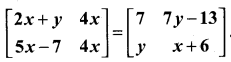Solution:
$$\left[\begin{array}{cc}{2 x+y} & {4 x} \\ {5 x-7} & {4 x}\end{array}\right]=\left[\begin{array}{cc}{7} & {7 y-13} \\ {y} & {x+6}\end{array}\right]$$
⇒ 2x + y = 7 ………….. (1)
4x = 7y – 13 ………….. (2)
5x – 7 = y …………… (3)
4x = x + 6 ……………. (4)
from (4) 4x – x = 6
3x = 6 ⇒ x = $$\frac{6}{3}$$ = 2
Substituting x = 2 in (1), we get
2(2) + y = 7 ⇒ 4 + y = 7 ⇒ y = 7 – 4 = 3
So x = 2 and y = 3
∴ x + y = 2 + 3 = 5

11th Maths 7.1 Exercise Question 4.
Determine the matrices A and B if they satisfy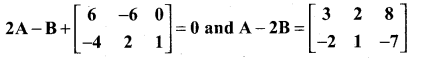Solution: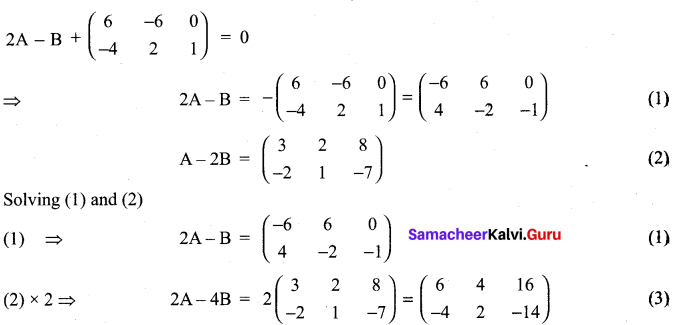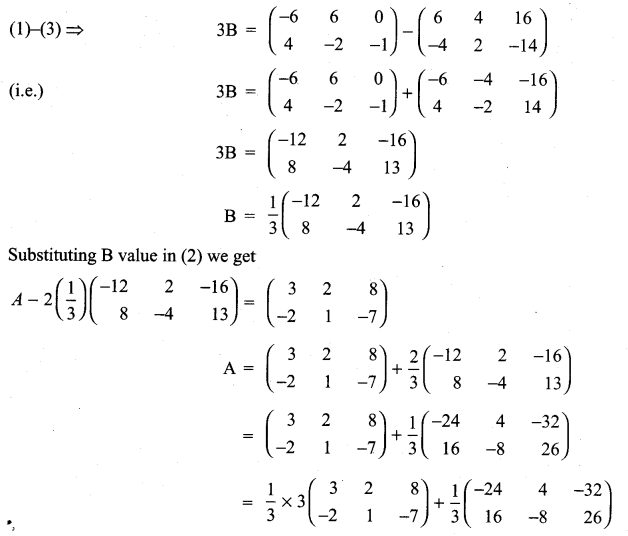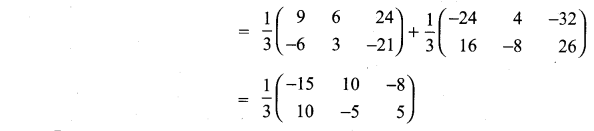11th Maths Volume 2 Exercise 7.1 Question 5.
If A = $$\left[\begin{array}{ll}{\mathbf{1}} & {\boldsymbol{a}} \\ {\mathbf{0}} & {\mathbf{1}}\end{array}\right]$$, then compute A4
Solution: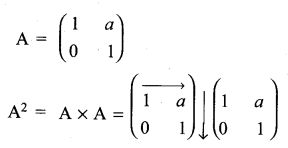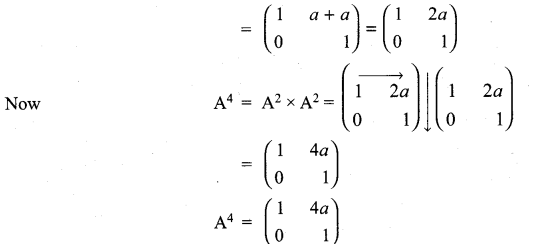11th Maths Matrix Solutions Question 6.
Consider the matrix Aα = $$\left[\begin{array}{cc}{\cos \alpha} & {-\sin \alpha} \\ {\sin \alpha} & {\cos \alpha}\end{array}\right]$$
(i) Show that AαAβ = Aα + β.
(ii) Find all possible real values of satisfying the condition Aα + ATα = 1.
Solution: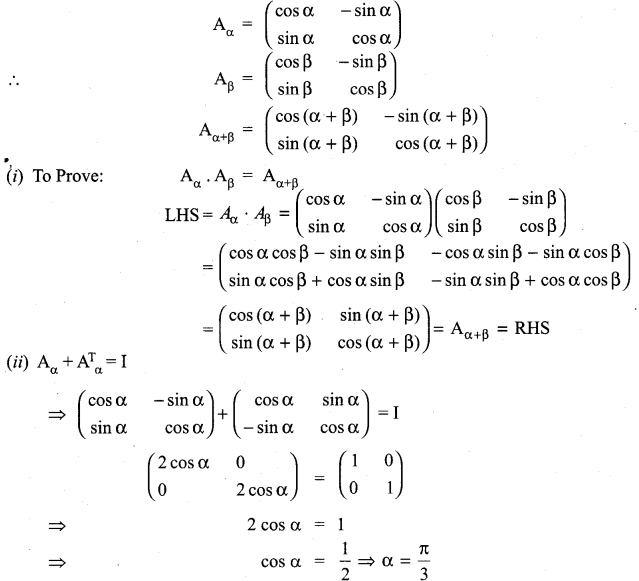General solution is α = 2nπ + $$\frac{\pi}{3}$$, n ∈ Z

11th Maths Exercise 7.1 Tamil Medium Question 7.
If A = $$\left[\begin{array}{rr}{4} & {2} \\ {-1} & {x}\end{array}\right]$$ such that (A – 2I) (A – 3I) = 0, find the value of x.
Solution: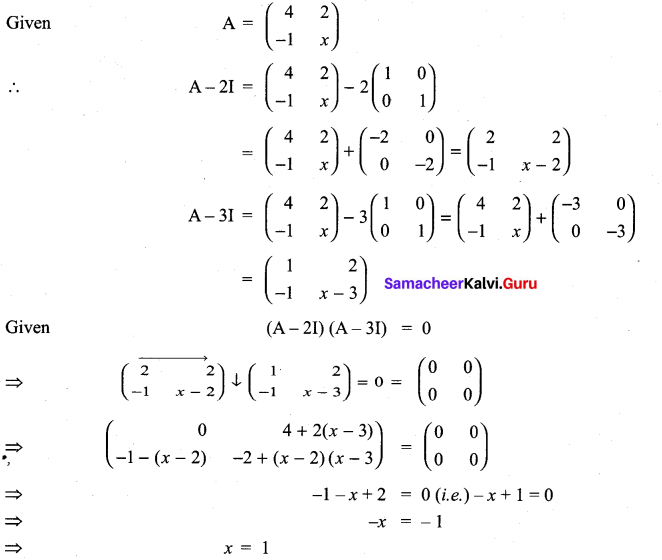11th Maths Matrices And Determinants Pdf Question 8.
If A = $$\left[\begin{array}{ccc}{\mathbf{1}} & {\mathbf{0}} & {\mathbf{0}} \\ {\mathbf{0}} & {\mathbf{1}} & {\mathbf{0}} \\ {\boldsymbol{a}} & {\boldsymbol{b}} & {-\mathbf{1}}\end{array}\right]$$, show that A2 is a unit matrix.
Solution: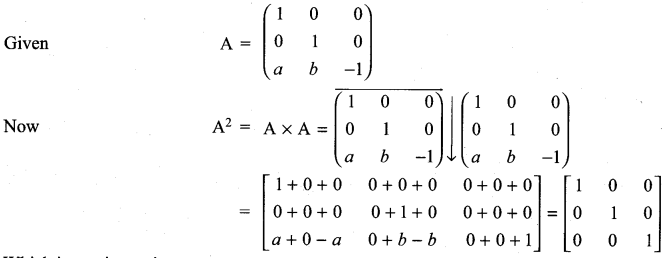Exercise 7.1 Class 11 Maths State Board Question 9.
If A =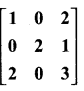and A3 – 6A2 + 7A + KI = 0, find the value of k.
Solution: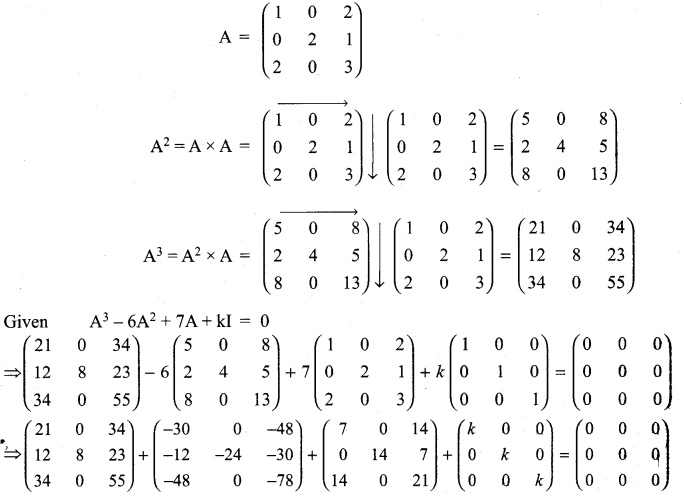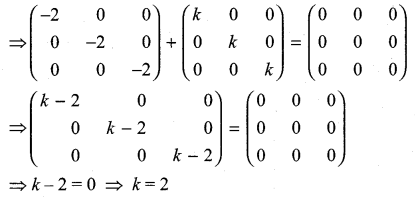11th Maths 7.1 Question 10.
Give your own examples of matrices satisfying the following conditions in each case:
(i) A and B such that AB ≠ BA.
(ii) A and B such that AB = 0 = BA, A ≠ 0 and B ≠ 0.
(iii) A and B such that AB = 0 and BA ≠ 0.
Solution: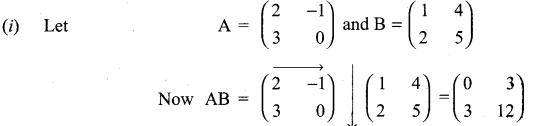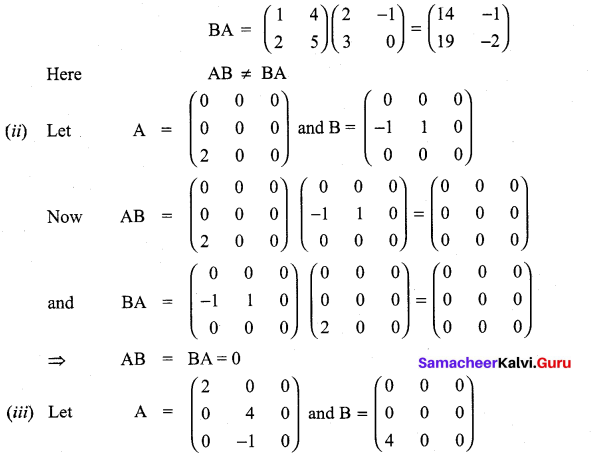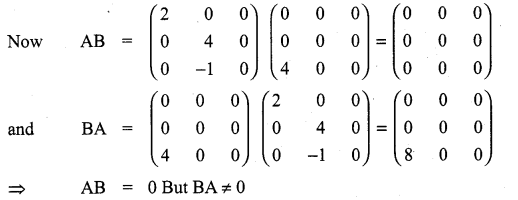11th Maths Matrix And Determinants Question 11.
Show that f(x) f(y) = f(x + y), where f(x) =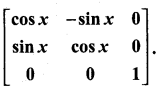Solution: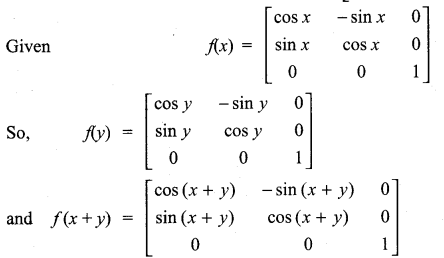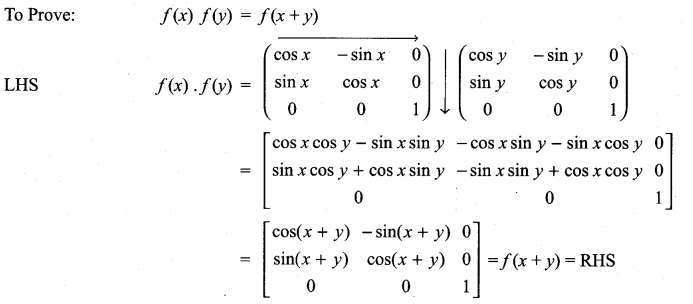11th Maths Exercise 7.1 In Tamil Question 12.
If A is a square matrix such that A2 = A, find the value of 7A – (I + A)3.
Solution:
Given A2 = A
So 7A – (I + A)3 = 7A – (I + 3A + 3A2 + A3]
= 7A – I – 3A – 3 A2 – A3
Given A2 = A
7A – I – 3A – 3A – A3 = -I + A – A3
= -I + A – (A2 × A)
= -I + A – (A × A) = -I + A – A2
= -I + A – A = -I
So the value of 7A – (I + A)3 = -I.

Question 13.
Verify the property A (B + C) = AB + AC, when the matrices A, B and C are given by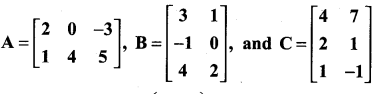Solution: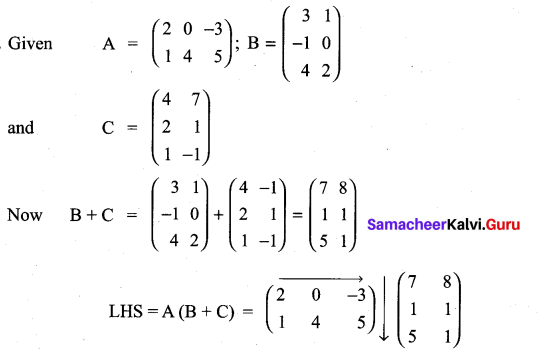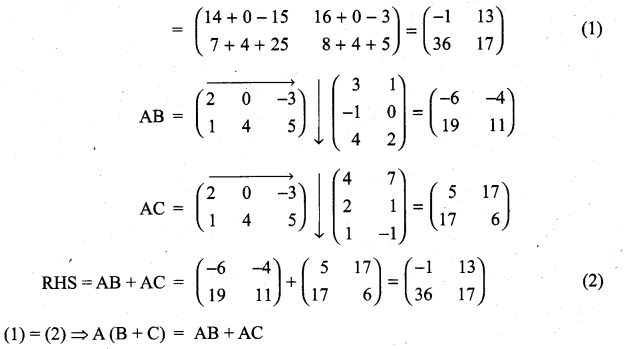11th Maths Volume 2 Exercise 7.1 Answers Question 14.
Find the matrix A which satisfies the matrix relation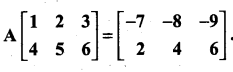Solution: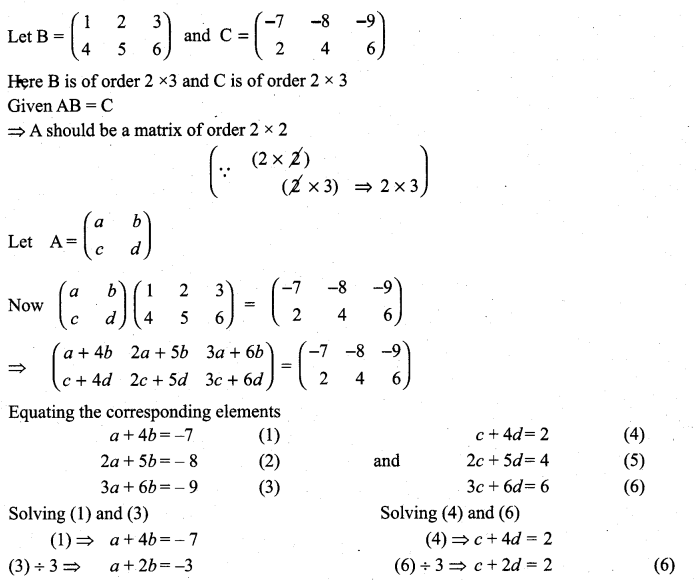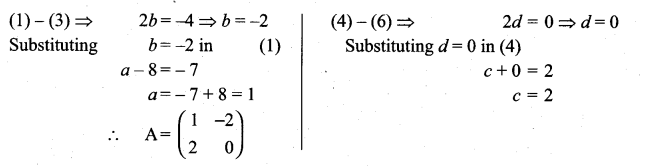11 Maths Exercise 7.1 Question 15.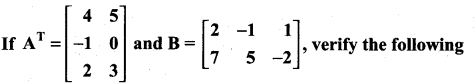(i) (A + B)T = AT + BT = BT + AT
(ii) (A – B)T = AT – BT
(iii) (BT)T = B.
Solution: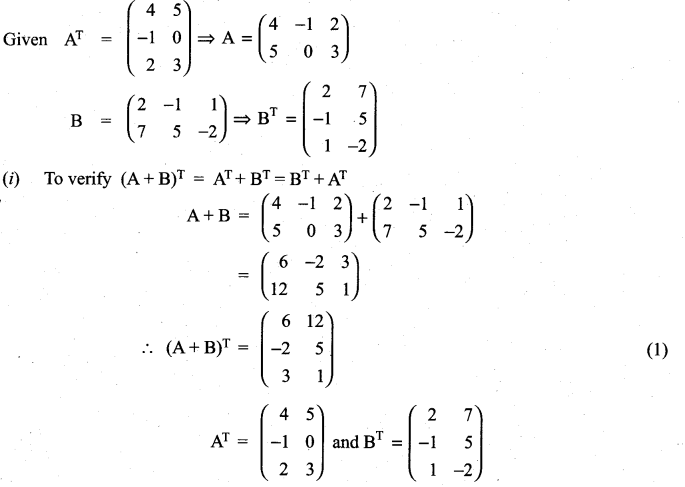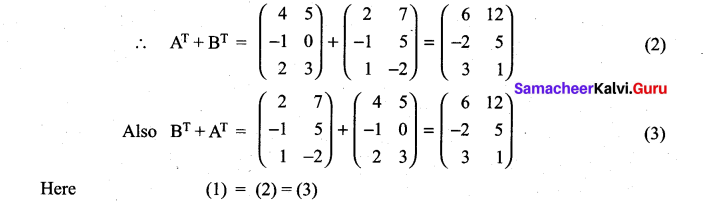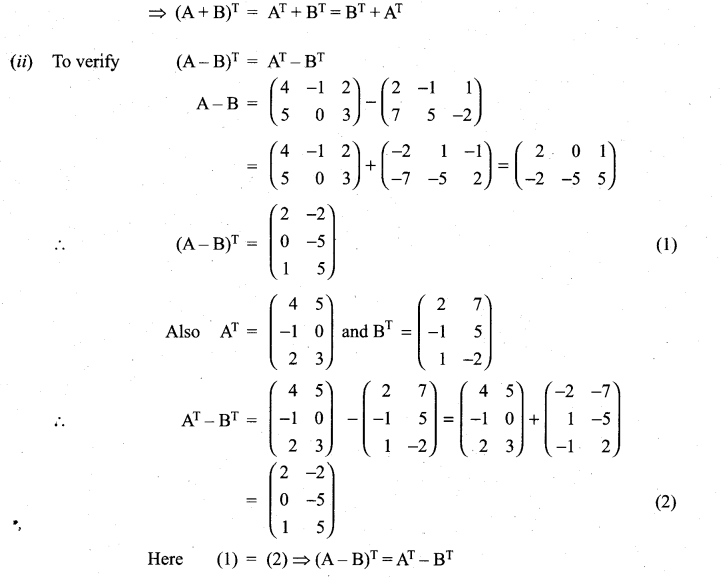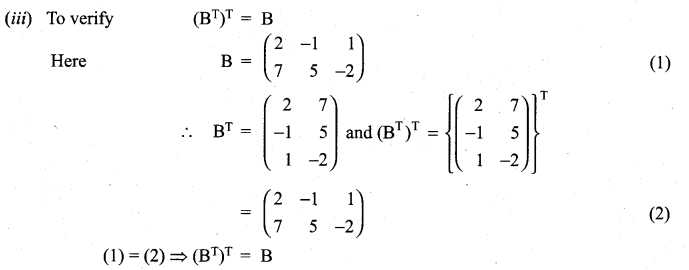11th Maths 7th Chapter Question 16.
If A is a 3 × 4 matrix and B is a matrix such that both ATB and BAT are defined, what is the order of the matrix B?
Sol.
A is a matrix of order 3 × 4
So AT will be a matrix of order 4 × 3
AT B will be defined when B is a matrix of order 3 × n
BAT will be defirted when B is of order m × 4
from (1) and (2) we see that B should be a matrix of order 3 × 4

Question 17.
Express the following matrices as the sum of a symmetric matrix and a skew-symmetric matrix: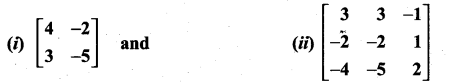Solution: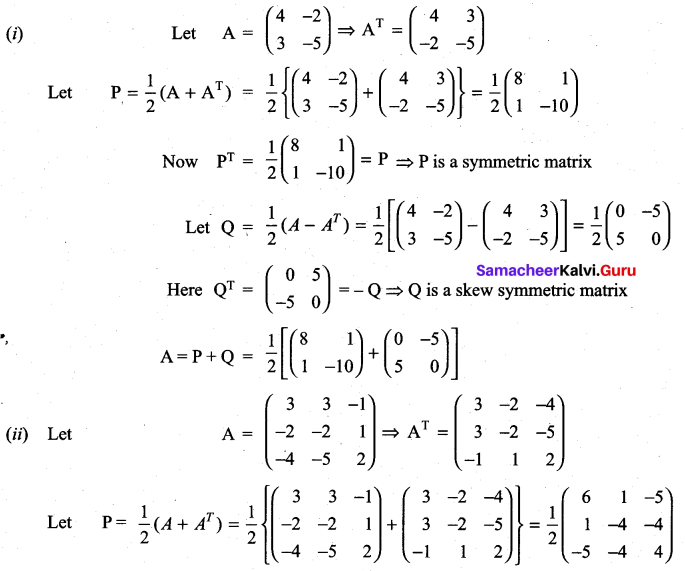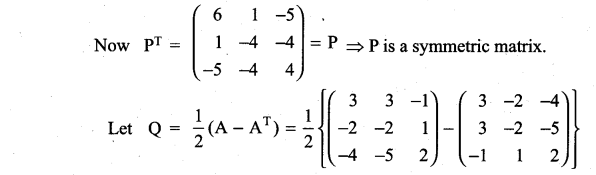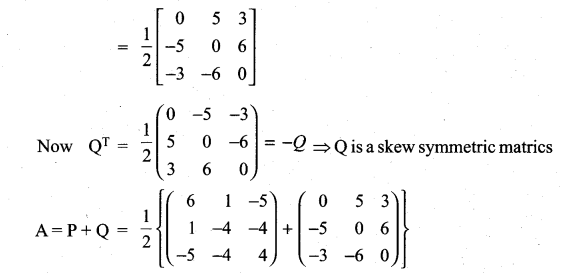Matrices And Determinants Class 11 State Board Solutions Question 18.
Find the matrix A such that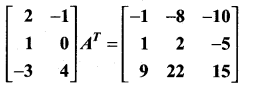Solution: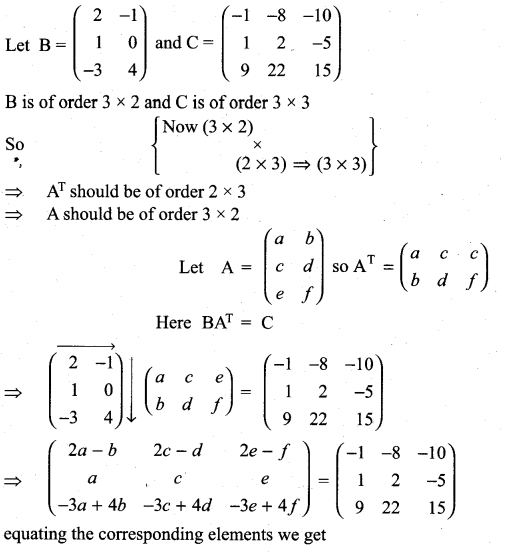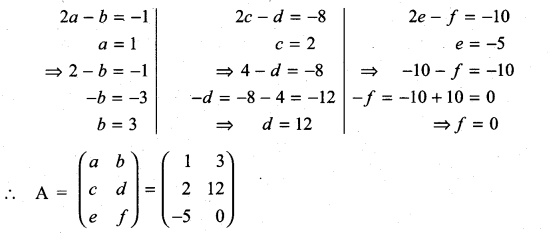11th Maths Determinants Solutions Question 19.
If A =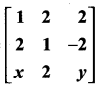is a matrix such that AAT = 9I, find the values of x and y.
Solution: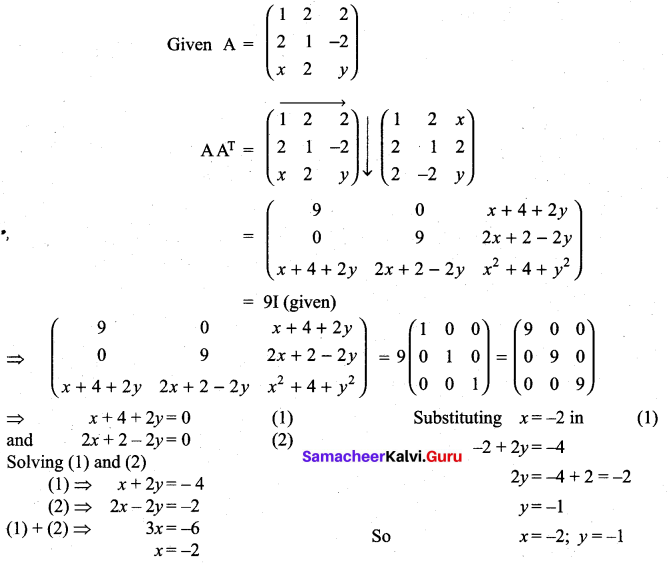Question 20.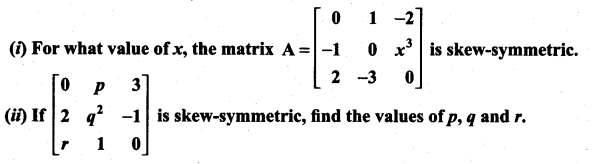Solution: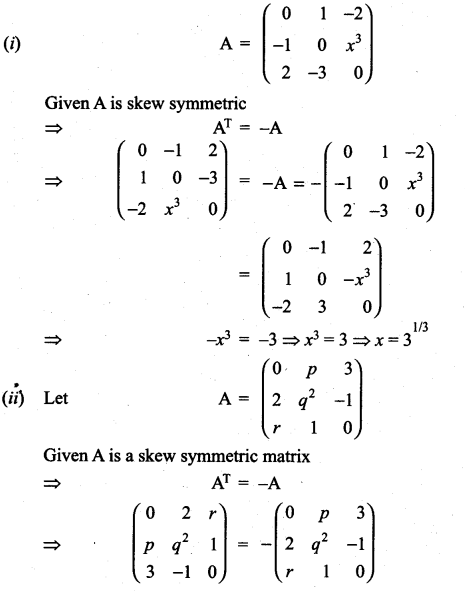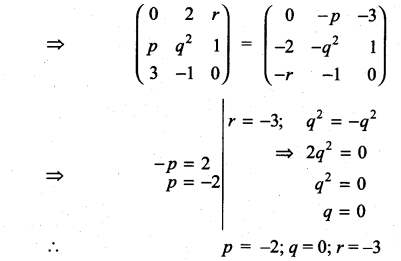Class 11th Maths Exercise 7.1 Question 21.
Construct the matrix A = [aij]3×3, where aij = i- j. State whether A is symmetric or skew- symmetric.
Solution:
Given A is a matrix of order 3 × 3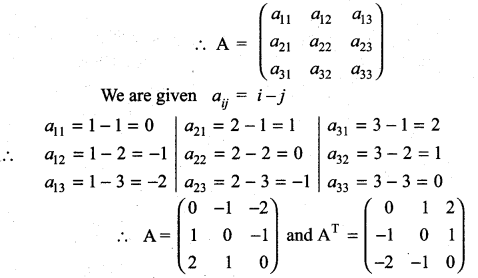Here AT = -A
⇒ A is skew symmetric

Matrices And Determinants Class 11 Solutions Pdf Question 22.
Let A and B be two symmetric matrices. Prove that AB = BA if and only if AB is a symmetric matrix.
Solution:
Let A and B be two symmetric matrices
⇒ AT = A and BT = B …………….. (1)
Given that AB = BA (2)
To prove AB is symmetric:
Now (AB)T = BTAT = BA
(from(1)) But (AB)T = AB by ………….. (2)
⇒ AB is symmetric.
Conversely let AB be a symmetric matrix.
⇒ (AB)T = AB
i.e. BTAT = AB
i.e. BA = AB (from (1))
⇒ AB is symmetric

Samacheer Kalvi 11th Maths Solutions Question 23.
If A and B are symmetric matrices of same order, prove that
(i) AB + BA is a symmetric matrix.
(li) AB – BA is a skew-symmetric matrix.
Solution:
Given A and B are symmetric matrices
⇒ – AT = A and BT = B
(i) To prove AB + BA is a symmetric matrix.
Proof: Now (AB + BA)T = (AB)T + (BA)T = BTAT + ATBT
= BA + AB = AB + BA
i.e. (AB + BA)T = AB + BA
⇒ (AB + BA) is a symmetric matrix.
(ii) To prove AB – BA is a skew symmetric matrix.
Proof: (AB – BA)T = (AB)T – (BA)T = BTAT – ATBT = BA – AB
i.e. (AB – BA)T = – (AB – BA)
⇒ AB – BA is a skew symmetric matrix.

11th Maths 7th Lesson Question 24.
A shopkeeper in a Nuts and Spices shop makes gift packs of cashew nuts, raisins and almonds.
Pack I contains 100 gm of cashew nuts, 100 gm of raisins and 50 gm of almonds. Pack-II contains 200 gm of cashew nuts, 100 gm of raisins and 100 gm of almonds. Pack-III contains 250 gm of cashew nuts, 250 gm of raisins and 150 gm of almonds. The cost of 50 gm of cashew nuts is ₹ 50, 50 gm of raisins is ₹ 10, and 50 gm of almonds is₹ 60. What is the cost of each gift pack?
Solution: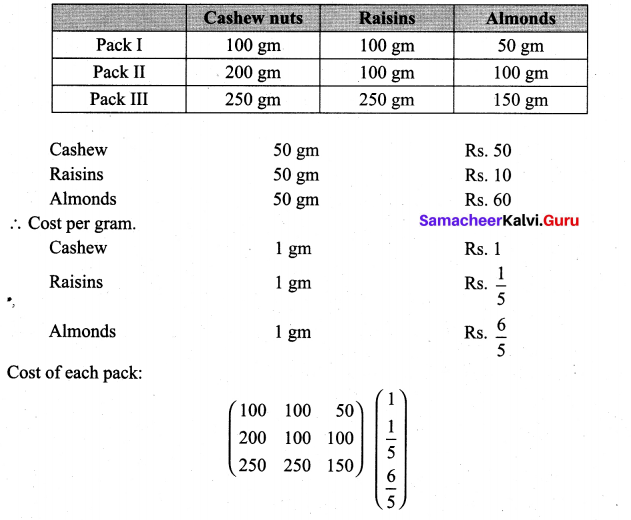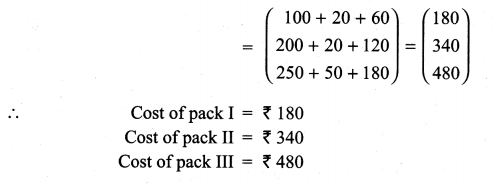### Samacheer Kalvi 11th Maths Solutions Chapter 7 Matrices and Determinants Ex 7.1 Additional Problems

Class 11 Maths Ex 7.1 Solutions Question 1.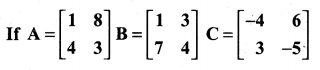Prove that (i) AB ≠ BA
(ii) A(BC) = (AB) C
(iii) A(B + C) = AB + AC
(iv) AI = IA = A
Solution: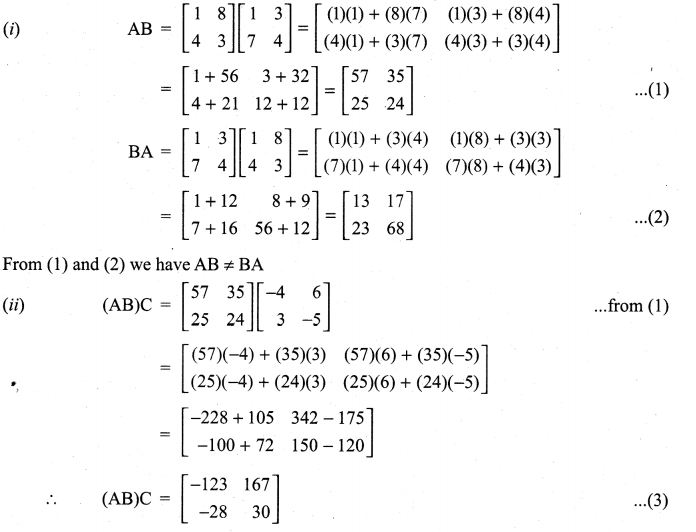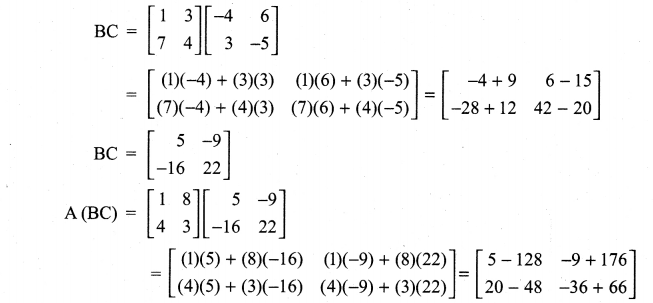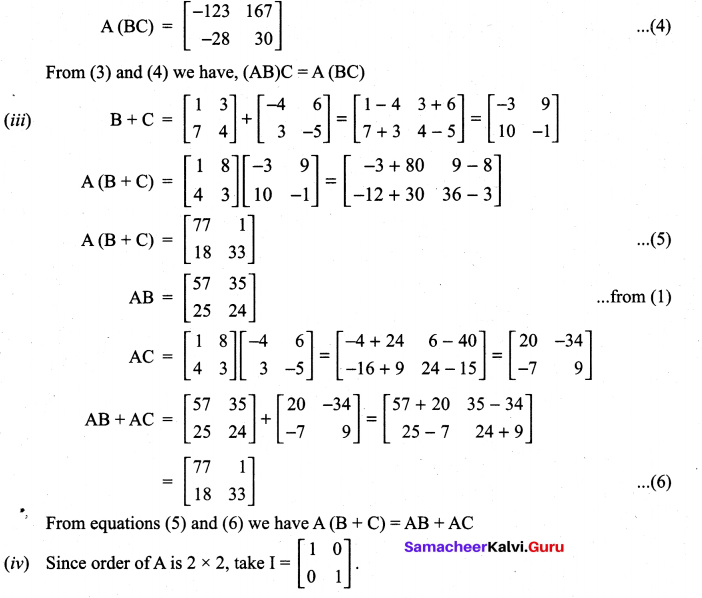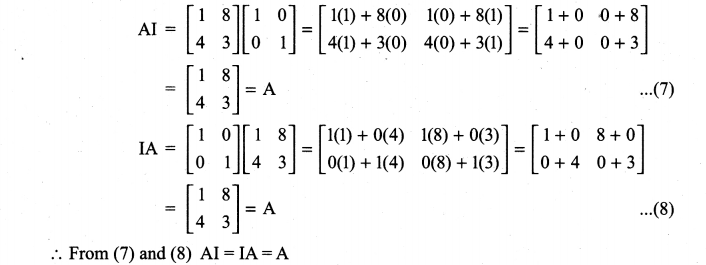Class 11th Maths Chapter 7 Exercise 7.1 Question 2.
If A = $$\left[\begin{array}{ll}{2} & {3} \\ {4} & {5}\end{array}\right]$$ find A2 – 7A – 2I.
Solution: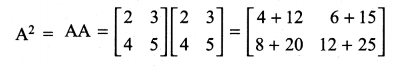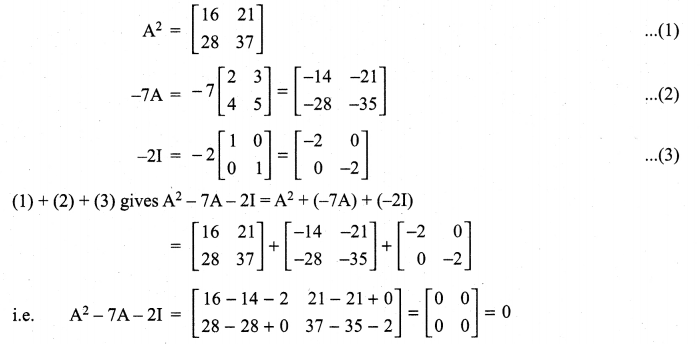11th Maths Guide Question 3.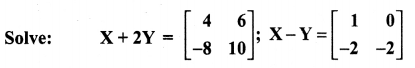Solution: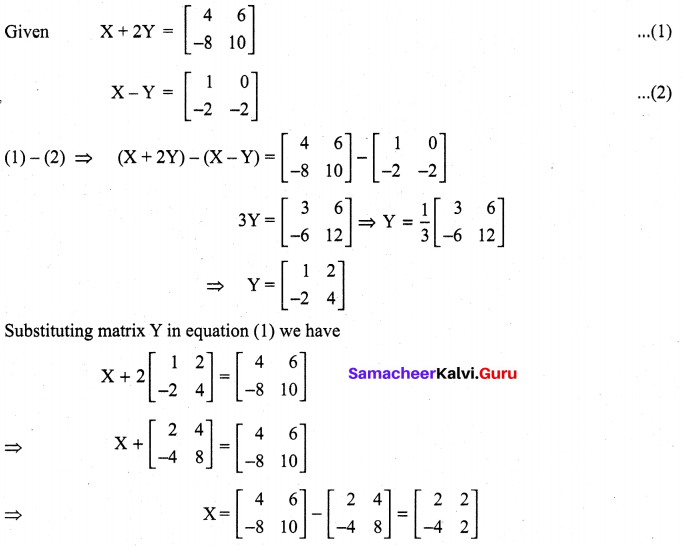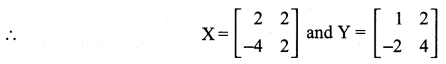11th Maths Guide Question 4.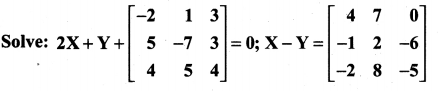Solution: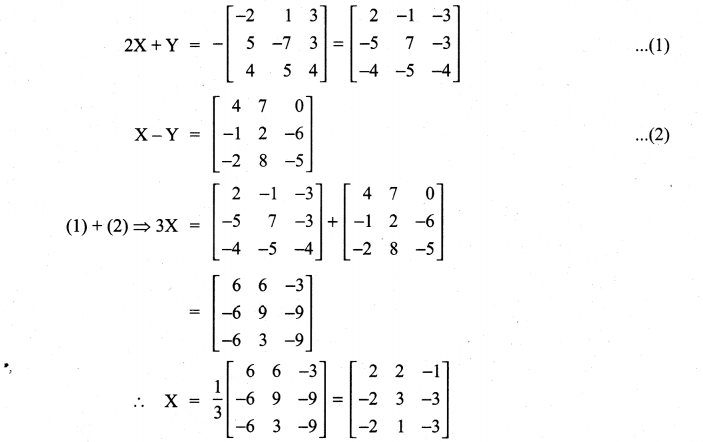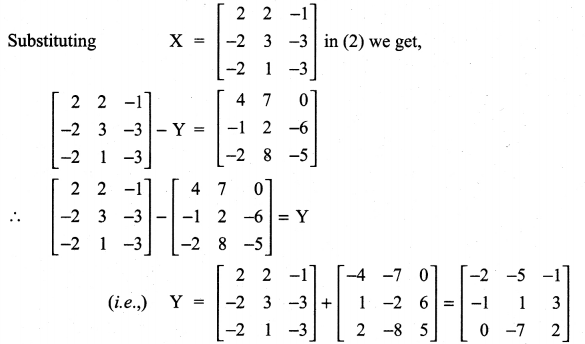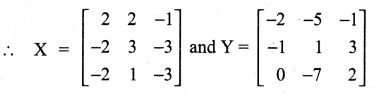Question 5.
If A = $$\left[\begin{array}{rr}{3} & {-5} \\ {-4} & {2}\end{array}\right]$$, show that A2 – 5A – 14I = 0 where I is the unit matrix of order 2.
Solution: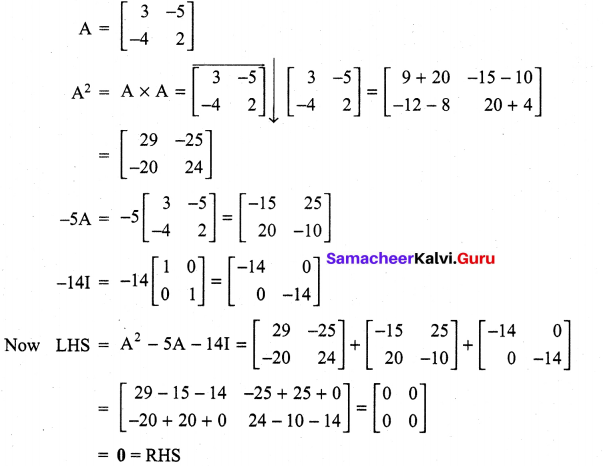Question 6.
If A = $$\left[\begin{array}{rr}{3} & {-2} \\ {4} & {-2}\end{array}\right]$$, find k so that A2 = kA – 2I
Solution: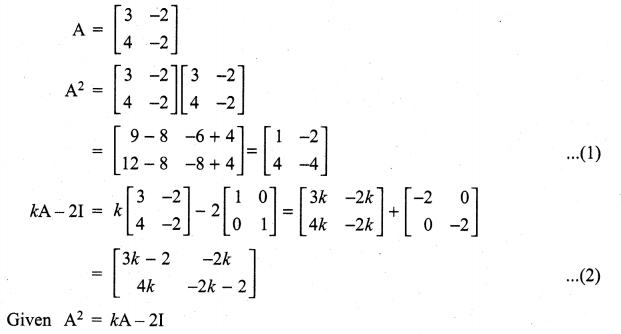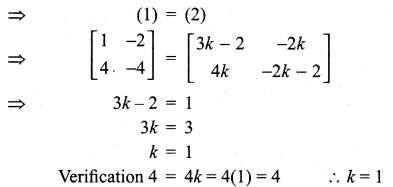Question 7.
If A = $$\left[\begin{array}{lll}{1} & {2} & {2} \\ {2} & {1} & {2} \\ {2} & {2} & {1}\end{array}\right]$$, show that A2 – 4A – 5I = 0
Solution: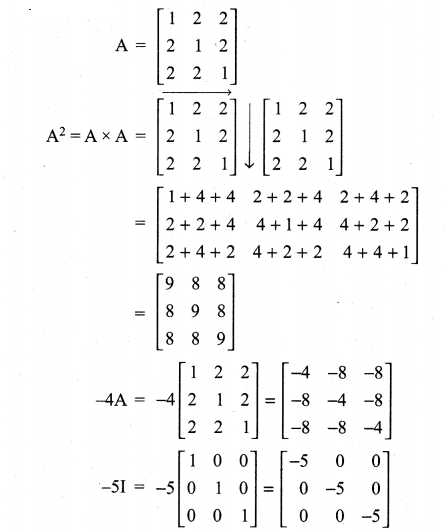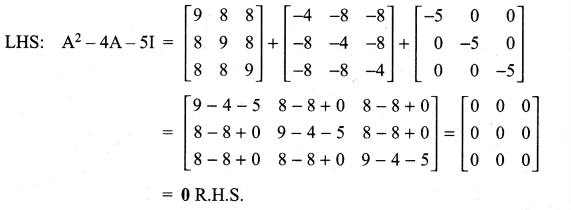Question 8.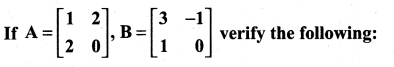Solution: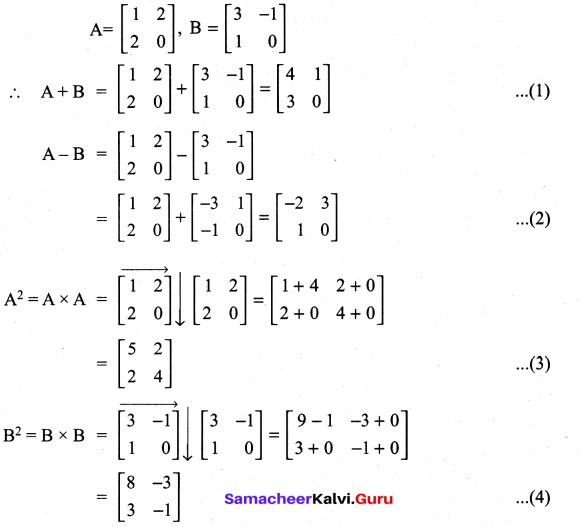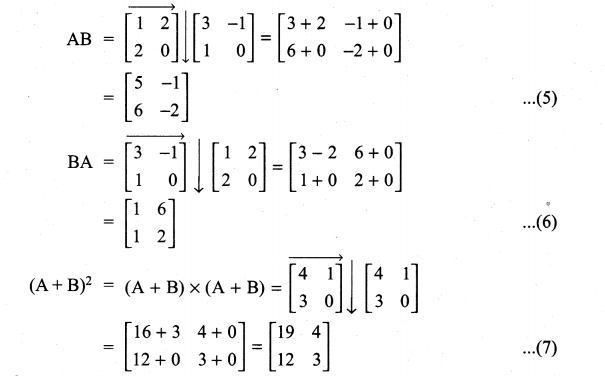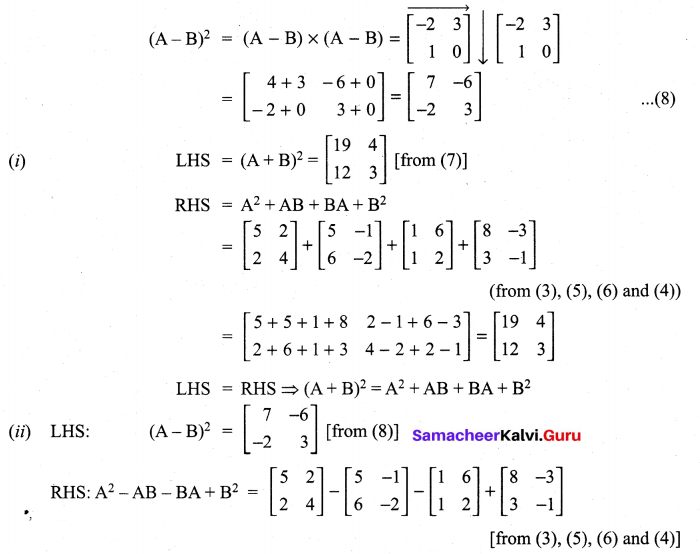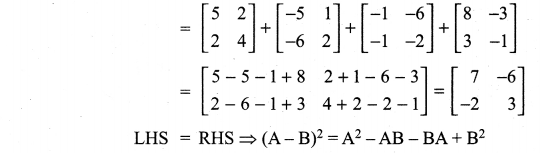Question 9.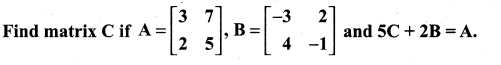Solution: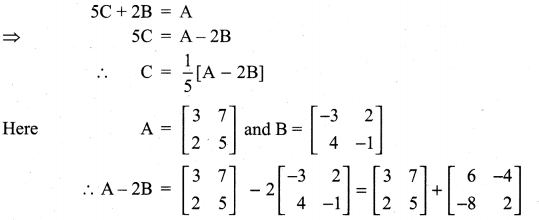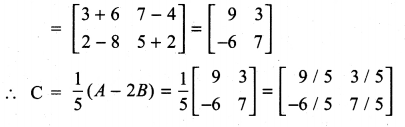Question 10.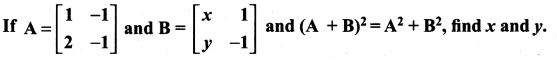Solution: